Courses

# Test: Transmission Lines- 2

## 20 Questions MCQ Test Topicwise Question Bank for Electronics Engineering | Test: Transmission Lines- 2

Description
This mock test of Test: Transmission Lines- 2 for Electronics and Communication Engineering (ECE) helps you for every Electronics and Communication Engineering (ECE) entrance exam. This contains 20 Multiple Choice Questions for Electronics and Communication Engineering (ECE) Test: Transmission Lines- 2 (mcq) to study with solutions a complete question bank. The solved questions answers in this Test: Transmission Lines- 2 quiz give you a good mix of easy questions and tough questions. Electronics and Communication Engineering (ECE) students definitely take this Test: Transmission Lines- 2 exercise for a better result in the exam. You can find other Test: Transmission Lines- 2 extra questions, long questions & short questions for Electronics and Communication Engineering (ECE) on EduRev as well by searching above.
QUESTION: 1

### Double stub matching eliminates standing waves on the

Solution:

Double stub matching eliminates standing waves on the source side of the left stub.

QUESTION: 2

### If Zsc = 64Ω and Zoc = 100 Ω, the characteristic impedance will be given by.

Solution:

Characteristic impedance is given by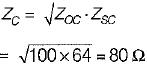QUESTION: 3

### Consider a lossless line with characteristic impedance R0 and VSWR = S. Then, the impedance at the point of voltage maxima and voltage minima are respectively given by

Solution:

Given,
Z0 = R0
we have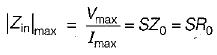and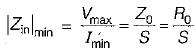QUESTION: 4

If K is the reflection coefficient and S is the voltage standing wave ratio, then

Solution:

Voltage standing wave ratio is given by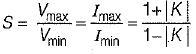or,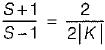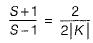(Using componendo and dividendo)
or,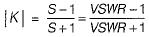QUESTION: 5

In terms of R, L, G and C the propagation constant of a transmission line is given by

Solution:

Propagation constant of a transmission line is given by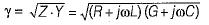QUESTION: 6

A line has  Z0 = 300∠0º Ω, and ZL = 150∠0º Ω  Voltage standing wave ratio (S) is given by

Solution:

Given
Z0 = 300∠0º
and ZL =150∠0ºΩ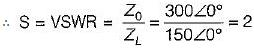QUESTION: 7

The characteristic impedance of a distortionless line is

Solution:

Characteristic impedance,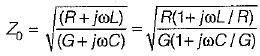or,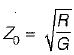(Since L/R = C/G for a distortionless line)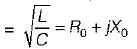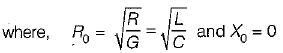Hence, characteristic impedance of a distortionless line is purely real.

QUESTION: 8

A transmission line works as a

Solution:
QUESTION: 9

A transmission line with a characteristic impedance Z0 is connected to a transmission line with characteristic impedance Zc. if the systme is being driven by a generator connected to the first line, then the overall transmission coefficient of current will be

Solution: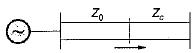The overall transmission coefficient is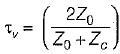QUESTION: 10

Which of the following statements related to a transmission line is/are correct?
1. Transmission line elements are integral parts of the antenna, in some antenna system.
2. A feeder is a particular case of a transmission Sine.
3. A lossless transmission line doesn’t has resistance but, has a non-zero value of leakage conductance.
4. At radio frequency (RF), R and G both are neglected.

Solution:
• Statements-1 and 2 are true.
• Statement-3 is not correct because in a lossless transmission line there is neither resistive loss (i.e. R = 0) nor any leakage current (i.e. G = 0).
• At radio frequency (RF), the inductive reactance is much larger than the resistance and so also the capacitie susceptance in comparison to the shunt conductance. Hence, at radio frequencies R and G both are neglected. Thus, statement-4 is correct.
QUESTION: 11

Assertion (A): A finite transmission line terminated in its characteristic impedance Z0, is equivalent to an infinite line.
Reason (R): The input impedance of an infinite line is the characteristic impedance of the line.

Solution:

The input impedance of a finite line terminated in its characteristic impedance (Z0), is equivalent to an infinite line because the input impedance of an infinite line is the characteristic impedance of the line (Z0). Hence, both assertion and reason are true and reason is the correct explanation of assertion.

QUESTION: 12

Consider the following statements:
1. Propagation constant is a dimensionless quantity.
2. When the line is lossless, propagation constant is directly proportional to the frequency.
3. Propagation constant when multiplied with the frequency gives the electrical length of the line.
Which of the above statements is/are true?

Solution:
• Propagation constant is dimensionless quantity because it is the ratio of voltages or currents.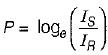or,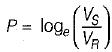(where, P = Propagation constant) Hence, statement-1 is correct.
• For a lossless transmission line,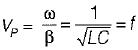∴ VP α f
Thus, statement - 2 is also correct.
• Statement-3 is also true.
Thus, all statements are true.
QUESTION: 13

Assertion (A): The group velocity is usually more than the phase velocity.
Reason (R): If the transmission line or transmission medium is such that different frequencies travel with different velocities, then the line or the medium is said to be dispersive.

Solution:

Group velocity is given by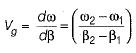Phase velocity or velocity of propagation is given by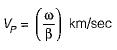The group velocity is usually less than the phase velocity. Hence, assertion is a false statement.

QUESTION: 14

Assertion (A): A transmission line act as resonant circuit and is used in many applications at high frequencies in antenna design and other ratio circuitory
Reason (R): An open and short-circuited lines behaves like resonant circuit when length of line is an integral multiple of λ/3.

Solution:

Assertion is correct because when a transmission line is open or short-circuited it behaves as resonant circuit. However, reason is false because this happens when length of the line is an integral multiple of λ/4.
We knnw that
Zoc = -j cot βl
and Zsc = jZ0 tan βl
Thus, when βl = length of line = nλ/4 , then
cot βl = 0
and tan βl = ∞
∴ Zoc = 0
and Zsc = ∞
This means a quarter wave short-circuit line represents an infinite impedance at inpul terminals, just like a parallel resonant (LC) circuil and a λ/4 open circuit line present zero impedance at input terminals just like a series resonant LC circuit.

QUESTION: 15

Assertion (A): Sometimes a quarter wave line is called as impedance inverter.
Reason (R): The quarter wavelength line transforms a load impedance ZR that is smaller than Z0 into a value Zs that is larger than Z0 and vice-versa.

Solution:
QUESTION: 16

ln an impedance smith chart, a clockwise movement along a constant resistance circle gives rise to

Solution:
QUESTION: 17

A transmission line whose characteristic impedance is purely resistive

Solution:
QUESTION: 18

The input impedance of a short circuited quarter wave long transmission line is

Solution:

Input impedance of a short-circuited quarter wave line depends on the nature of load impedance ZL.

QUESTION: 19

A rectangular air filled waveguide has cross section of 4 cm x 10 cm. The minimum frequency which can propagate in the waveguide is

Solution: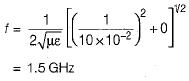QUESTION: 20

Consider the transmission system shown below: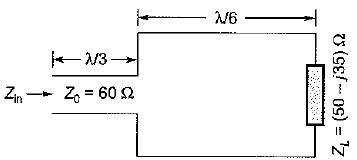The input impedance Zin is given by

Solution: